Next

<

Exact relations between critical exponents for elastic stiffness and electrical conductivity of percolating networks

David J. Bergman, School of Physics and Astronomy
Raymond and Beverly Sackler Faculty of Exact Sciences
Tel Aviv University, IL-69978 Tel Aviv, Israel

December 4, 2001

Abstract:

It has long been known that the critical exponent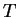of the elastic stiffness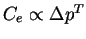of a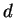-dimensional percolating network (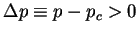measures the closeness of the network to its percolation threshold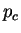) satisfies the following inequalities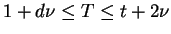, where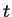is the critical exponent of the electrical conductivity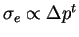of the same network and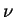is the critical exponent of the percolation correlation length. Similarly, the critical exponents which characterize the divergences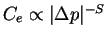,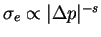of a percolating rigid/normal network (i.e., a random mixture of normal elastic bonds and totally rigid bonds) and a percolating superconducting/normal network (i.e., a random mixture of normal conducting bonds and perfectly conducting bonds;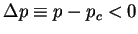now measures the closeness of the rigid or superconducting constituent to its percolation threshold) have long been known to satisfy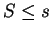. I now show that, when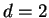or,is in fact exactly equal to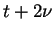and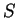is exactly equal to. This is achieved by a judicious use of some variational principles for electrical and elastic networks, and by a judicious treatment of constraints and short range correlations in those networks. An extension of these proofs to arbitrary (integer) values of the dimensionalityshould be possible.